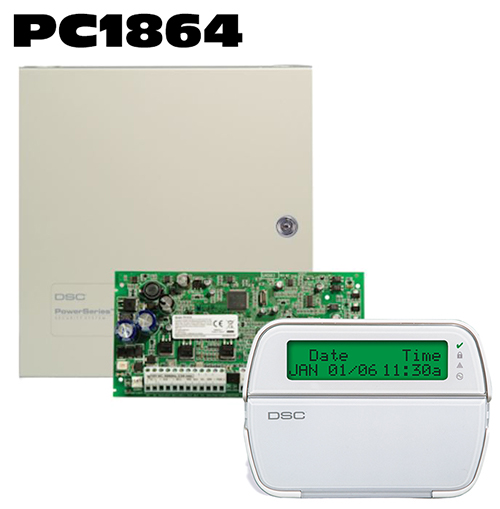In order to set the time and date on the DSC Powerseries PC1864 system, you will need to know the Master arming and Disarming code for the system. You will need to follow these steps Enter *6 + Master User Code + 1 + Time in Military Format + 2 digit month + 2 digit day + 2 Digit Year + #

Military time for the PC1864 system is as follows :

00:00 = 12am
01:00 = 1am
02:00 = 2am
03:00 = 3am
04:00 = 4am
05:00 = 5am
06:00 = 6am
07:00 = 7am
08:00 = 8am
09:00 = 9am
10:00 = 10am
11:00 = 11am
12:00 = 12pm
13:00 = 1pm
14:00 = 2pm
15:00 = 3pm
16:00 = 4pm
17:00 = 5pm
18:00 = 6pm
19:00 = 7pm
20:00 = 8pm
21:00 = 9pm
22:00 = 10pm
23:00 = 11pm

DSC Pc1864 Alarm Monitoring Services

DSC PC 1864 Frequently Asked Questions

DSC Pc1864 Alarm Monitoring Options